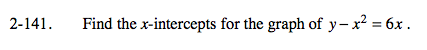### Home > CAAC > Chapter 13 > Lesson 13.RE2-S > Problem2-141

2-141.

Find the x-intercepts for the graph of y x2 = 6x. Homework Help ✎The x-intercepts are where y = 0.

Set the equation equal to 0 and use the Zero Product Property.